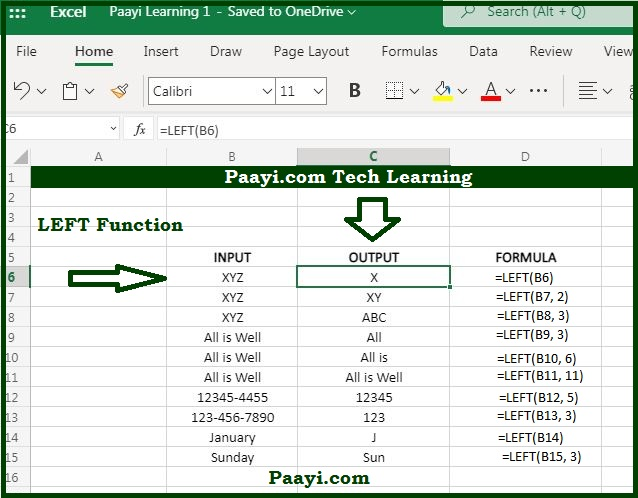# Learn How to Use Microsoft Excel LEFT Function

Written by | 0 Comments | 687 Views

In this article, you will learn how to use the Microsoft Excel LEFT function and its prime function in Microsoft Excel. You will also get to know the Microsoft Excel LEFT function return value and syntax with the help of some examples.

## Microsoft Excel LEFT Function

The main objective of the Microsoft Excel LEFT function is to extract text from the string's left side. So, with the help of the LEFT function, you can able to return the given number of characters from the left side of the text string provided. Let's say, for example:

= LEFT("apple", 3) -  the return value will be "app."

### LEFT Function Return Value

The return value will be the text from the left side of the string.

### LEFT Function Syntax

= LEFT(text, [num-chars])

Where the arguments:

• text: This is the text from which you want to extract the strings.
• num-chars: This is the number of characters you want to extract from a text provided.

## How to Use Microsoft Excel LEFT Function?As we know-how, you can use the LEFT function to extract the left side characters of the text provided. And the num-chars control the number of characters you want to extract. In case the num-chars is not provided, the default value will be 1. And if the num-chars is greater than the characters in the text provided, it will return the entire text.

In the same way, you can also use the RIGHT function to extract text starting from the right side of the text and the MID function to extract text from the middle of the text provided.

You can also combine the LEFT function with LEN and FIND function to perform more complex operations. Let us go to some of the examples given below:

Example 1: = LEFT("January", 3) - The return value will be "Jan".

Example 2: = LEFT("ABC") - The return value will be "A."